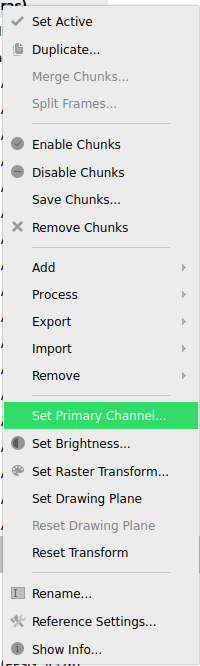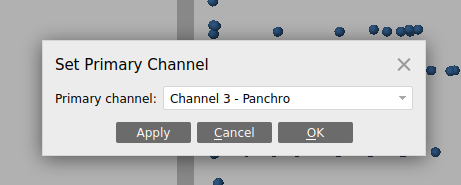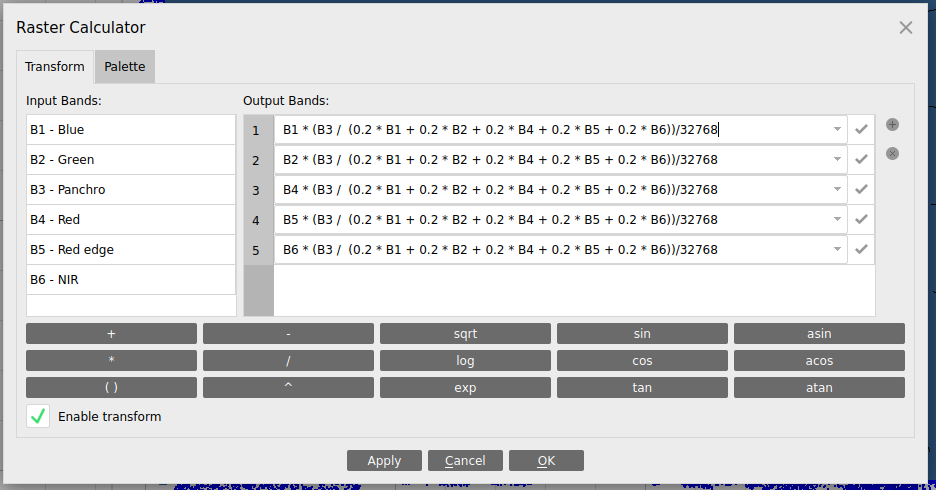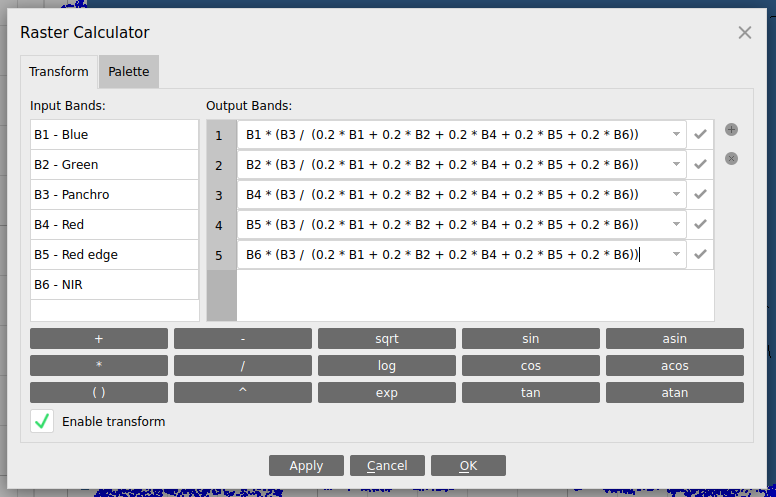# Pan-sharpening/processing data from Altum-PT and RedEdge-P cameras in Agisoft Metashape

There are only a few extra steps when processing data from panchromatic enabled cameras compared to processing RedEdge-MX or Altum data. For the general guide, view our guide here: Process MicaSense sensor data in Agisoft Metashape.

Before aligning the data, be sure to set the primary channel to be the Panchro channel under the Tools>Set Primary Channel menu:After changing this setting, you can continue to process as usual.

At the end of your usual processing workflow, you can use the Raster Calculator (Tools>Set Raster Transform to create a pan-sharpened version of your dataset. If you want reflectance values, you can divide all of the formulas by 32768, as seen below.

For the RedEdge-P:If you would prefer digital number values, you can use the formulas without the extra division:For Altum-PT:

The Altum-PT add the 7th band for the thermal or LWIR sensor. This band needs to be converted from centi-Kelvin to Celsius as described here and shown below.The formulas above can be copy pasted from here:

```#divide by 32768 to get the reflectance values for each band instead of digital numbersB1 * (B3 /  (0.2 * B1 + 0.2 * B2 + 0.2 * B4 + 0.2 * B5 + 0.2 * B6))/32768
B2 * (B3 /  (0.2 * B1 + 0.2 * B2 + 0.2 * B4 + 0.2 * B5 + 0.2 * B6))/32768
B4 * (B3 /  (0.2 * B1 + 0.2 * B2 + 0.2 * B4 + 0.2 * B5 + 0.2 * B6))/32768
B5 * (B3 /  (0.2 * B1 + 0.2 * B2 + 0.2 * B4 + 0.2 * B5 + 0.2 * B6))/32768
B6 * (B3 /  (0.2 * B1 + 0.2 * B2 + 0.2 * B4 + 0.2 * B5 + 0.2 * B6))/32768
#0.2 being default weight(B7 / 100) - 273.15    #Thermal band, Altum-PT only```

Finally, when you export the orthomosaic, you want to export this raster transform data by choosing "Index value" under the "Raster transform" option. If you don't want to create a pan-sharpened dataset, you can choose "None" for "Raster transform".When you are doing calculations (whether in GIS or within Metashape itself), the band order will now be the same as with a RedEdge or Altum:

 Band 1 Blue Band 2 Green Band 3 Red Band 4 Red edge Band 5 NIR Band 6 Thermal (LWIR) *Altum-PT only

If you want to create an RGB composite, you would use bands 3/2/1,  as you would use for a RedEdge-MX or Altum sensor. Likewise, NDVI would be:

(Band 5 - Band 3)/(Band 5 + Band 3)

To process in Pix4Dfields, see this article: Pan-sharpening RedEdge-P and Altum-PT data in Pix4Dfields